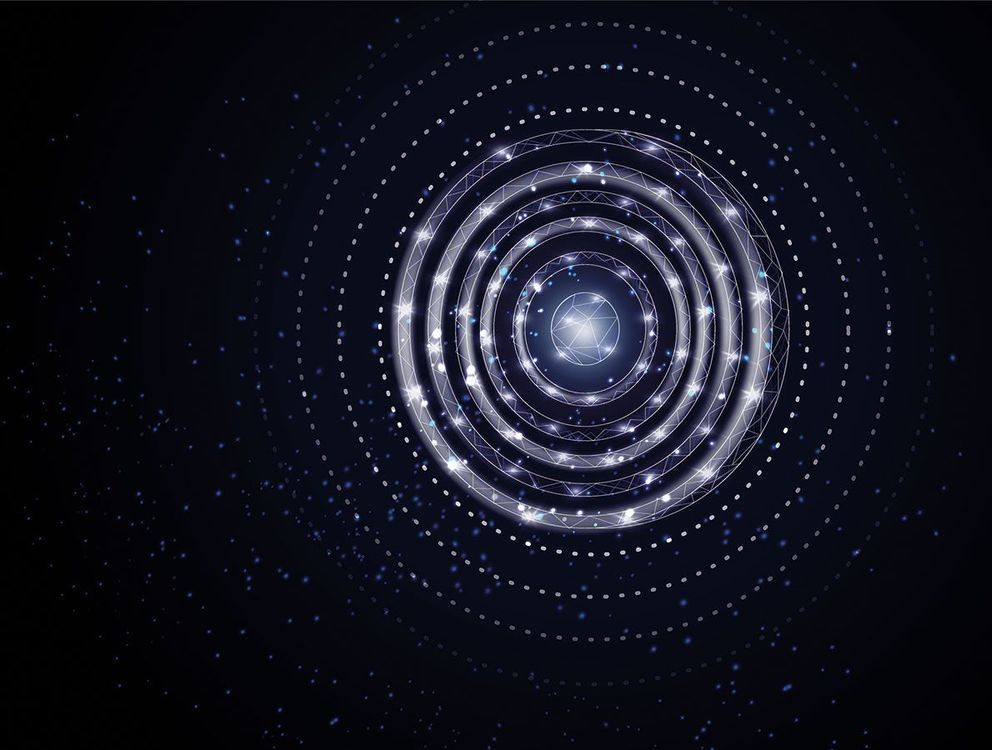## BlogCan we realize non-trivial condensed-matter phases – such as topological insulating phases – in the time dimension? Topological insulators are condensed-matter systems that are insulators in their interior but, by virtue of the topological properties of the electronic structure, have conducting surface (edge) states. They are characterized by global topological invariants. An example of a topological invariant is the number of holes a surface has: a sphere has no holes while a torus has one. It is hard to change such a topological invariant because it is not possible to gradually introduce a hole in a sphere in order to change it to a torus – either there is a hole or there is no hole, but there is nothing like a fraction of a hole. Even the vacuum (empty space) has trivial topological invariants. In order to reconcile a change of this invariant at the interface between the vacuum and a topological insulator, there are surface (edge) states that close the gap between the energy bands of the insulator, thereby producing conducting behaviour.

Can a quantum swing behave like an electron in a topological insulator? Yes, for example if we ask the child to push with a combination of a resonant frequency ω and a sub-harmonic frequency ω /2 (Optica 5 1390, New J. Phys. 21 052003). Then the motion of the swing effectively creates a chain of lattice sites along the resonant orbit with staggered hopping amplitudes, and thus reproduces an example of a topological system, called the Su–Schrieffer–Heeger lattice. In order to observe the edge states, we need to create an “edge” in the motion of the swing and then check if there are quantum states that are localized close to it. How can we create an edge in time? We ask the child to jump on the swing from time to time, which introduces a barrier in the chain of lattice sites along the resonant orbit and consequently breaks the time-translational symmetry along the orbit, similar to how a surface breaks spatial-translational symmetry in an ordinary topological insulator.